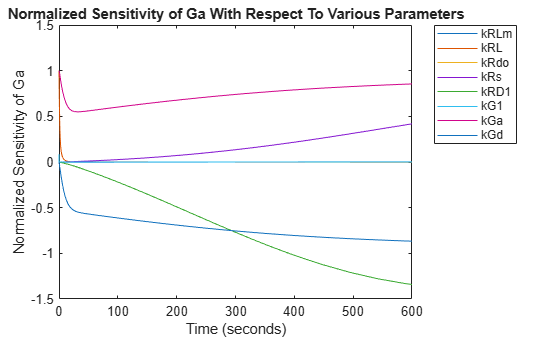## Calculate Sensitivities Using `sbiosimulate`

### Overview

This example uses the model described in Model of the Yeast Heterotrimeric G Protein Cycle to illustrate SimBiology® sensitivity analysis options.

This table lists the reactions used to model the G protein cycle and the corresponding rate parameters (rate constants) for each mass action reaction. For reversible reactions, the forward rate parameter is listed first.

No.NameReaction1Rate Parameters
1Receptor-ligand interaction`L + R <-> RL``kRL`, `kRLm`
2Heterotrimeric G protein formation`Gd + Gbg -> G``kG1`
3G protein activation`RL + G -> Ga + Gbg + RL``kGa`
4Receptor synthesis and degradation`R <-> null``kRdo`, `kRs`
5Receptor-ligand degradation`RL -> null``kRD1`
6G protein inactivation`Ga -> Gd``kGd`
1 Legend of species: L = ligand (alpha factor), R = alpha-factor receptor, Gd = inactive G-alpha-GDP, Gbg = free levels of G-beta:G-gamma complex, G = inactive Gbg:Gd complex, Ga = active G-alpha-GTP

Assume that you are calculating the sensitivity of species `Ga` with respect to every parameter in the model. Thus, you want to calculate the time-dependent derivatives

$\frac{\partial \left(Ga\right)}{\partial \left(kRLm\right)},\frac{\partial \left(Ga\right)}{\partial \left(kRL\right)},\frac{\partial \left(Ga\right)}{\partial \left(kG1\right)},\frac{\partial \left(Ga\right)}{\partial \left(kGa\right)}...$

### Load and Configure the Model for Sensitivity Analysis

1. The `gprotein_norules.sbproj` project contains a model that represents the wild-type strain (stored in variable `m1`).

`sbioloadproject gprotein_norules m1`
2. The options for sensitivity analysis are in the configuration set object. Get the configuration set object from the model.

`csObj = getconfigset(m1);`
3. Use the `sbioselect` function, which lets you query by type, to retrieve the `Ga` species from the model.

`Ga = sbioselect(m1,'Type','species','Where','Name','==','Ga');`
4. Set the `Outputs` property of the `SensitivityAnalysisOptions` object to the `Ga` species.

`csObj.SensitivityAnalysisOptions.Outputs = Ga;`
5. Use the `sbioselect` function, which lets you query by type, to retrieve all the parameters from the model and store the vector in a variable, `pif`.

`pif = sbioselect(m1,'Type','parameter');`
6. Set the `Inputs` property of the `SensitivityAnalysisOptions` object to the `pif` variable containing the parameters.

`csObj.SensitivityAnalysisOptions.Inputs = pif;`
7. Enable sensitivity analysis in the configuration set object (`csObj`) by setting the `SensitivityAnalysis` option to `true`.

`csObj.SolverOptions.SensitivityAnalysis = true;`
8. Set the `Normalization` property of the `SensitivityAnalysisOptions` object to perform `'Full'` normalization.

`csObj.SensitivityAnalysisOptions.Normalization = 'Full';`

### Perform Sensitivity Analysis

Simulate the model and return the data to a ```SimData object```:

`simDataObj = sbiosimulate(m1);`

### Extract and Plot Sensitivity Data

You can extract sensitivity results using the `getsensmatrix` method of a `SimData object`. In this example, `R` is the sensitivity of the species `Ga` with respect to eight parameters. This example shows how to compare the variation of sensitivity of `Ga` with respect to various parameters, and find the parameters that affect `Ga` the most.

1. Extract sensitivity data in output variables `T` (time), `R` (sensitivity data for species `Ga`), `snames` (names of the states specified for sensitivity analysis), and `ifacs` (names of the input factors used for sensitivity analysis):

`[T, R, snames, ifacs] = getsensmatrix(simDataObj);`
2. Because `R` is a 3-D array with dimensions corresponding to times, output factors, and input factors, reshape `R` into columns of input factors to facilitate visualization and plotting:

`R2 = squeeze(R);`
3. After extracting the data and reshaping the matrix, plot the data:

```figure; plot(T,R2); title('Normalized Sensitivity of Ga With Respect To Various Parameters'); xlabel('Time (seconds)'); ylabel('Normalized Sensitivity of Ga'); leg = legend(ifacs, 'Location', 'NorthEastOutside'); set(leg, 'Interpreter', 'none');```From the previous plot you can see that `Ga` is most sensitive to parameters `kGd`, `kRs`, `kRD1`, and `kGa`. This suggests that the amounts of active G protein in the cell depends on the rate of:

• Receptor synthesis

• Degradation of the receptor-ligand complex

• G protein activation

• G protein inactivation

## SupportGet trial now